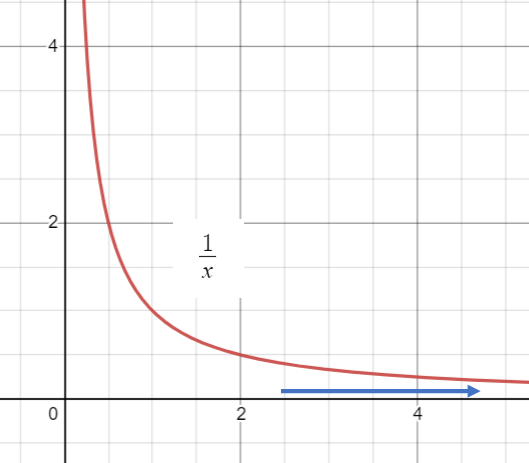# Integral Diverges / Converges: Meaning, Examples

When an integral diverges, it fails to settle on a certain number or it’s value is ±infinity. More formally, we say that a divergent integral is where an improper integral’s limit doesn’t exist. On the other hand, if the limit is finite and that limit is the value of the improper integral, the integral is convergent .

To put this in plain English, the term “integral diverges” means that you can’t calculate the area under a curve. For example, the following graph shows the function f(x) = 1/x:Let’s say you wanted to find the area from x = 1 to x = ∞ (your bounds of integration). You can’t calculate a number because as the graph travels to infinity (blue arrow), the area will constantly increase a little more. When the trouble spot is at the end of the graph (more formally called an endpoint), it’s called a simple improper integral .

## How to Show if an Integral Diverges

Improper integrals aren’t that useful in calculus; When you come across one, the first step is usually to replace it with a proper integral (one with defined limits of integration).In the above image, the improper integral on the left can’t be evaluated. To find out if the integral diverges:

• Replace the improper integral with a limit of a proper integrals.
• Find the limit (if it exists).

If the limit doesn’t exist, the integral diverges .

## Examples

Example problem #1: Does the following improper integral diverge?Step 1: Replace the improper integral with a limit of a proper integrals:Step 2: Find the limit:The limit is a finite number (1), so this integral converges.

Example problem #2: Does the following improper integral diverge?Step 1: Replace the improper integral with a limit of a proper integrals:Step 2: Find the limit:The limit is infinite, so this integral diverges.

The integral test is used to see if the integral converges; It also applies to series as well. If the test shows that the improper integral (or series) doesn’t converge, then it diverges.

## References

Graph: Desmos.com.
 Blair, R. Improper Integrals (With Solutions). Retrieved April 7, 2021 from: https://www2.math.upenn.edu/~ryblair/Math104/papers/Lec3_12Sol.pdf
 Swenton, F. (2009). Overview of Improper Integrals. Retrieved April 7, 2021 from: https://web.math.princeton.edu/~nelson/104/ImproperIntegrals.pdf
 Wakefield, N. et al. 5.10 Improper Integrals. Retrieved April 7, 2021 from: https://mathbooks.unl.edu/Calculus/sec-5-10-improper.html

CITE THIS AS:
Stephanie Glen. "Integral Diverges / Converges: Meaning, Examples" From StatisticsHowTo.com: Elementary Statistics for the rest of us! https://www.statisticshowto.com/integral-diverges-converges/